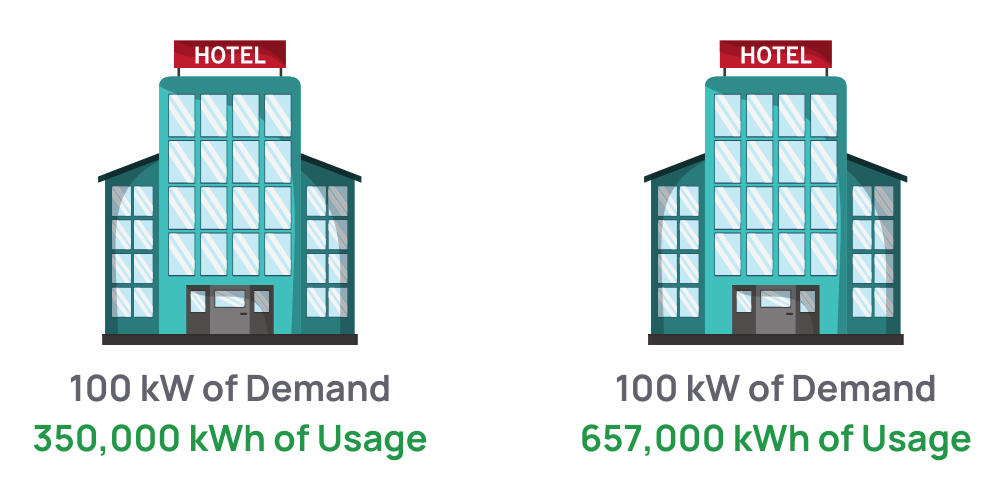#### At a Glance:

Understanding Load Factor (LF) is critical to being a successful energy broker. In the retail energy space, LF is an important metric when reviewing electricity pricing. High LF means lower rates from suppliers, which translates to lower prices for your customers.

Electric Load Factor is a calculation used in the retail energy business to quickly determine the cost profile (cost to serve) for a particular account based on their energy consumption (kWh) in relation to their demand (kW).  LF is generally measured using a 12 month analysis of Consumption and Demand (see below).

To better understand how it works, let’s take a closer look at the two main components we use to calculate LF.

• Energy Consumption: How much electricity was used over a period of time. We measure this in kWh (usage)
• Energy Demand/Capacity: What was the most amount of energy being used at any given moment. We measure this in kW (demand)

A simple calculation using these two components can give you a good view into a customer’s usage profile, and is a quick way to determine what they can expect to pay for their electricity supply.  Simply stated, LF is a measurement of the amount of energy used over a period of time in relation to the highest demand in that same period.

Let’s review a real life scenario using two seemingly “identical” hotels.An electricity supplier pricing these 2 hotels will have the same cost for capacity despite the fact that each hotel uses a different amount of kWh’s. Let’s assume the following for each component:
Supplier Costs for Hotel 1
• Electricity = 350,000 kWh x \$0.035 = \$12,250
• Capacity = 100 kW x \$0.20 x 365 days = \$7,300
• Total Cost = \$19,550 / 350,000kwh = \$0.0558 per kWh
Supplier Costs for Hotel 2
• Electricity = 657,000 kWh x \$0.035 = \$22,995
• Capacity = 100 kW x \$0.20 x 365 days = \$7,300
• Total Cost = \$30,295 / 657,000kwh = \$0.0461 per kWh

Although Hotel 2 is using more kWh’s, its total cost per kWh is less. Since the capacity costs between both hotels are identical (based on 100 kW of demand), the supplier can spread those costs across more kWh’s with Hotel 2, hence, lowering the total price per kwh.

## How Is Load Factor Calculated?

Now let’s review the actual LF calculation using the example above. We will see that Hotel 2, which has a cheaper rate per kWh, has the higher LF, and Hotel 1 has a lower LF. Remember, LF is a measurement of the amount of energy used over a period of time in relation to the highest demand in that same period. In the Hotel example we are measuring the annual usage over 12 months (or 8760 hours which is the total hours in a year).

• Hotel 1 = 350,000 kWh / (100 kW * 8760) = 40% LF
• Hotel 2 = 657,000 kWh / (100 kW * 8760) = 75% LF

## Why Is Load Factor Important?

Load Factor is important in retail electricity pricing for so many reasons. First, it is a quick way for suppliers to determine their total costs for a particular customer without having to manually calculate each component of the electricity rate. Also, Load Factor allows energy brokers a way to determine where and how to price their customers. Typically, customers with high Load Factor ratings (above 50%) should always be custom priced with suppliers. Others, with lower ratings can be placed on a supplier matrix.

## How Can I Calculate Load Factor On My Own?

In order to accurately calculate Load Factor the same way the suppliers do, you need certain data from your customer or their utility company. In many markets, costs for capacity are calculated based on peak demand averages in the summer and a kW rating (or capacity tag) is assigned to the customer’s account each year. In order to understand the actual Load Factor % for your customer, you need that data. Luckily, Diversegy has access to that data and tools to help you calculate Load Factor

A simple way to roughly estimate a customer’s load factor is to use their peak kW reading from the highest summer month and perform the calculation found above. Typically, utility companies will insert charts on electric bills that have this data.

# Become an Energy Partner.

## What are the Key Benefits of Diversegy’s Sales Partner Program?

Diversegy’s full-serve sales platform offers agents, teams, brokers, and customers many benefits. Our program is a commitment and investment into the future of the competitive retail energy industry. Here are some of the key benefits of our platform:

# Get in touch with us today.

• This field is for validation purposes and should be left unchanged.

# Get in touch with us today.

• This field is for validation purposes and should be left unchanged.